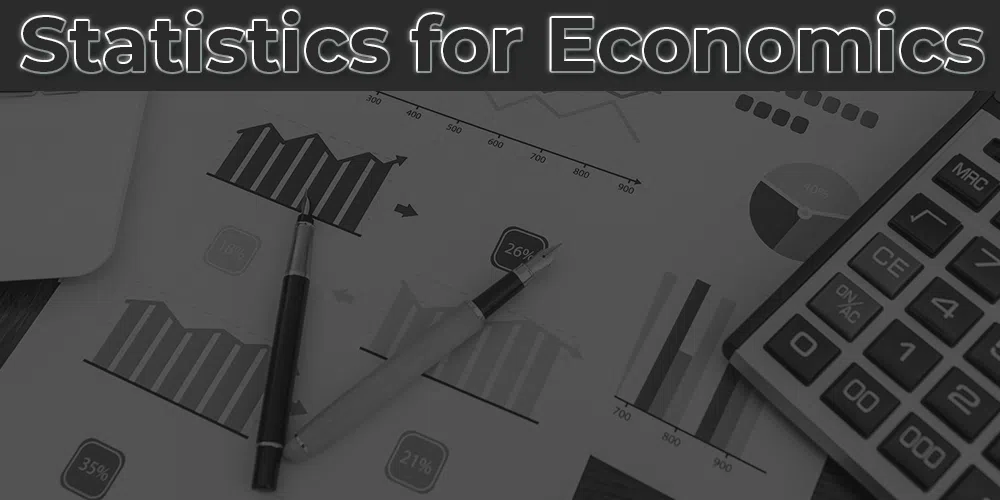Open In App
Related Articles

# Statistics for Economics

The word Statistics is derived from the Greek word ‘Statistique,’ the Latin word ‘Status,’ the Italian word ‘Statista,’ and the German word ‘Statistic.’ Statistics is defined as the study, collection, analysis, interpretation, and organization of data for different ultimate objectives. Statistics help a user in gathering and analyzing huge numerical data easily and efficiently. Statistics can be easily defined in two senses: Plural Sense and Singular Sense.

Statistics for Economics plays a major role in economics. Statistics helps in the study of market structure and understanding the different economic problems. After a better understanding of the economic problems, statistics also help in solving those issues by formulating appropriate economic policies. Every economics branch takes the help of statistics to prove different economic theories. One can also establish a mathematical relationship with the help of statistics. Economists can present the facts of economics precisely.## CBSE Class 11 Statistics for Economics

### Chapter 1: Concept of Economics and Significance of Statistics in Economics

1. Introduction to Statistics for Economics

### Chapter 10: Index Number

#### Important Formulas:

Important Formulas in Statistics for Economics